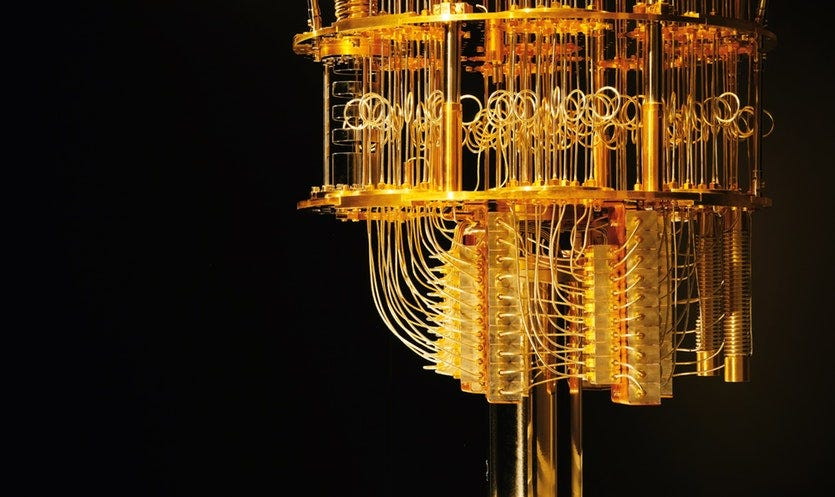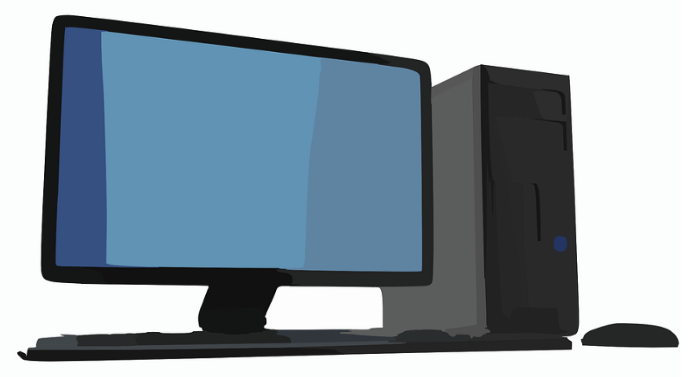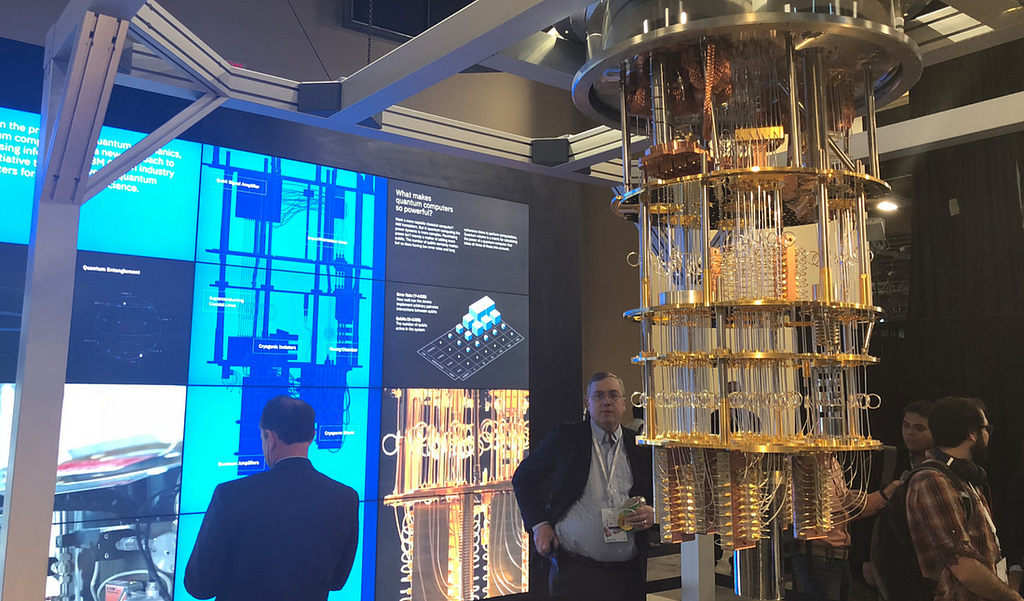# Quantum Computing Explained – By Shaan Ray### Introduction

​Advances in computing require appropriate hardware. Though computers have become smaller and more powerful over time, the power of regular computers (also known as classical computers) is limited.

​Quantum computers are a new generation of computers built to solve the problem of exponential scaling (for example, finding the optimal solution to a problem in which there are too many possibilities for a classical computer to analyze).

### Background on Classical Computers

Classical computers have many components (including main memory, arithmetic unit, control unit, and others). They represent, process, and control data through these components. Computer chips contain modules, which include logic gates, which in turn include transistors.

A computer module is a collection of electronic circuits on a circuit board. The logic gates are tiny computers within the computer itself. They look at two bits and push one of them out as an output. Their job is to read any input, in order to produce an output. A transistor is a switch that either allows or denies information to pass through it. The combinations of the logic gates form modules that allow for the basic functions of a computer. If we think of the transistor as an electric switch, the electricity is moving from one place to another when the switch is on. If the switch is off, then electrons are blocked.

Computer components are getting smaller. A typical scale for transistors today is 14 nanometers, which is 500 times smaller than a red blood cell. As these transistors decrease in size, electrons can move to the other side of a blocked passage, resulting in it not being blocked at all. This process is called ‘quantum tunneling’, and it is slowing down our technological progress.Our computers are based on a binary system (also known as a base 2 numeral system), which uses 0 and 1 as bits. A bit, derived from ‘binary unit’, is a unit of information for a computer that holds the values of 0 or 1. For example, a 64-bit computer can work with 64 binary numbers at a time.

Combinations of these bits are used to represent more complex data and operations. The logic gate performs a Boolean function, producing a single binary output. Boolean logic is a division of algebra that is used to create true and false statements. Since classical computers operate in binary, their logic is expressed in Boolean terms. The computer uses operators such as AND, OR and NOT to express the value and return a true or false output. For example, if you have values for x and y and the logical expression states x AND y, the computer would return true if both are true, while if it said x OR y, it would return true if at least one were true.

An easy programming example, minus the programming language would be:

x = 2, y = 4

x AND y are greater than 1: this would return true.

x OR y are greater than 2: this would also return true, as y is greater than 2.

In a classical computer, a true statement could (for example) return a value of 1, while a false statement could return a value of 0. This forms the basis of classical computing: though most calculations require more than one simple true/false statement, classical computers are large combinations of these binary statements. This is what everything from clicking a mouse to opening a browser rests on.

### What Are Quantum Computers

For certain problems that a classical computer solves, you may need a very small number of logic gates. However, if you want to find the factors of an extremely large number, it is going to take a large magnitude of logic gates (which a classical computer will not have).

A quantum bit, known as a qubit, is a computer bit that is able to hold two different states at once, meaning that it can hold a position of 0 and 1 at the same time. A regular bit can only hold one of the two at a specific time.

A qubit can be any two-level quantum system — a spin and a magnetic field, or a single photon (particle representing a quantum of light). This system’s possible states are 0 and 1. Within the quantum realm, the qubit can be in any proportion of both states at once; this phenomenon is called superposition.

Superposition allows quantum computers to analyze far more possibilities than a classical computer. As soon as you test the value of the photon by sending it through a filter, it needs to decide on vertical or horizontal polarization. You cannot predict if it will decide on position 0 or 1. When in superposition, the photon is in some combination of both 0 and 1 simultaneously. However, as soon as you measure its value, it collapses into one of the defined states.An exhibit of an IBM quantum computer.

### Numerical Example

​Think of 4 bits that can be on or off. Such a system would have 2⁴ (so, 16) possible combinations. In a traditional setting, you can use only one of these. However, qubits could hold all of these 16 combinations at once. This number grows exponentially with every added qubit.

### Entanglement

​Qubits can hold the property of ‘entanglement’, a close connection between qubits that allows them to react to a change in the other’s state instantly no matter how far apart they are. Therefore, when measuring one entangled qubit, you can directly conclude the properties of its partner without having to test it.

### ​Qubit Manipulation

​As a traditional logic gate receives a simple set of inputs, it produces one definite output. The quantum gate manipulates an input of superpositions, rotates probabilities, and finally produces a determined state as its output. To break down the steps, a quantum computer:

1. Sets up qubits
2. Applies qubit gates to entangle them and manipulate probabilities
3. Measures the outcome, collapsing the qubits into one defined state: a sequence of 0s and 1s. This means no more superposition.

This way, all calculations in the setup are done at the exact same time.

### Simplistic Overview

​The essential power of a quantum computer is that you can consider many states simultaneously. In order to make it work, its algorithm must be able to produce an end state that is readable (so, the information that you read out at the end cannot have superpositions). This means that quantum computers require a more complex algorithm design to be useful.

### Where Quantum Computers are Effective

Quantum computers will most likely not replace our home computers. However, they can be superior in areas such as data searching. To find something in a database, a classical computer must test every one of its entries. A quantum computer will take the square root of that time to come up with the same answer.

Quantum computers can challenge existing IT security measures. Currently, data is protected by various levels of encryption. In this case, you can give everyone a public key to encode messages only you can decode. While technically this public key can be used to calculate your secret private key by the use of trial and error on a classical computer, it would take far too long to be worth anyone’s time. A quantum computer with exponentially higher speed will be able to do it much faster.

### Two conceptual explanations of how quantum computers work

Example 1

You have a 10 person dinner party. You need to figure out how to seat everyone. There are 10! = 3,628,800 ways of doing so (where ‘10!’ is pronounced ‘ten factorial’ and represents 10 x 9 x 8 x…x 1. A classical computer will have to go through each of the 3.6 million ways individually and then compare them to figure out the best optimization.

​A quantum computer would:

1. Take the qubits and go into a superposition of all possible states and configurations
2. When this problem is encoded into a quantum computer, the encoder applies a phase on each of the states. When the ways are in phase, the amplitudes add. When they are out of phase, the amplitudes cancel. This is a similar idea to noise cancelling headphones, which create noise to phase out outer noise.
3. You use interference to amplify some answers, while cancelling the others, finally approaching one answer.

Example 2

Here we have a maze. Imagine you are in the center of it and want to get out at either of the two exits. You can start exploring each path, one by one. After a lot of tries, you will finally get out of the maze.Now, imagine you have with you several clones of yourself. Everyone can start exploring the different ways, and one clone will directly find the correct way out. You and your clones were exploring all the different paths at once, meaning that you were all in different places at the same time. You were in a superposition of states, like a qubit. This allows you to find the best solution possible, quickly.

These simplified, conceptual examples help explain why quantum computers (when created) will be immensely helpful in solving large, complex problems.